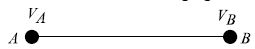Physics

# Explain on Potential Difference

Volt: If the work is done in bringing 1 coulomb (1C) of positive charge from infinity to a point in the electric field is 1 joule (1J), then the potential at that point is called 1 volt (1V).

The potential at a point in an electric field is 20V means to bring 1 coulomb (1C) positive charge from infinity to that point 20J work is to be done.Potential difference: Let, in an electric field A and B are two points and the potentials of the points are VA and VB respectively (fig). The work done in bringing a unit positive charge from infinity to point A is VA and to point B is VB. Therefore the work done in bringing a unit positive charge from point B to point A is VA –VB i.e. the potential difference between these two points

The work done in transferring a unit positive charge from one point to another point in an electric field is called the potential difference between two points.